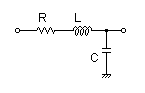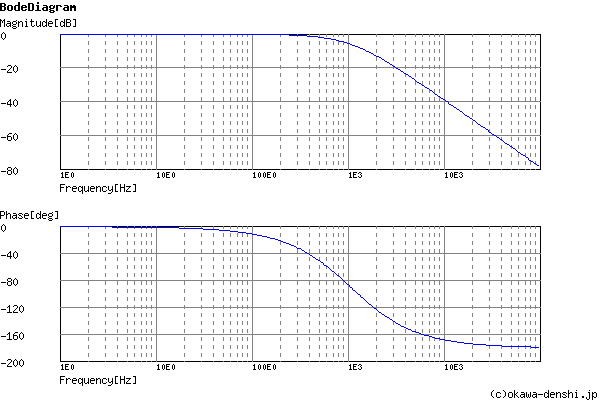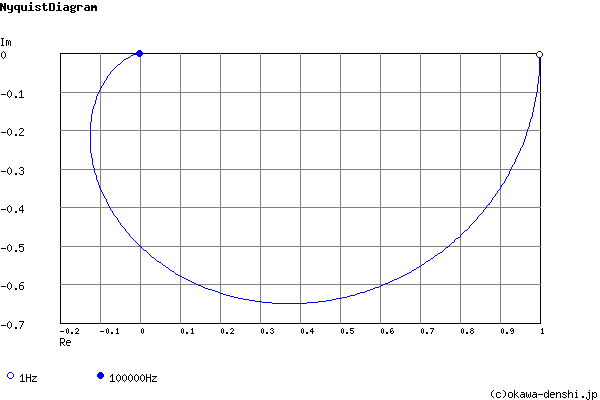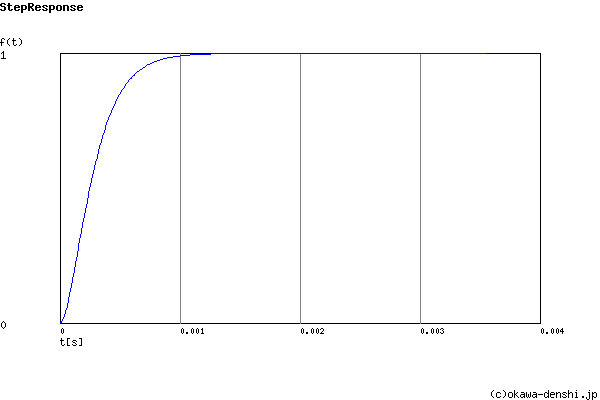# (Sample)RLC Low-pass Filter Design Tool - Result -

Calculated the transfer function for the RLC Low-pass filter, displayed on graphs, showing Bode diagram, Nyquist diagram, Impulse response and Step response.

## RLC Filter

 Vin(s)→→Vout(s)
(Sample)Transfer Function:
 G(s)= 45454545.4545 s2+13483.9972493s+45454545.4545

R = 1.34839972493Ω
C = 220uF
L = 100uH

#### Cut-off frequency

fc = 1073.02240743[Hz]

Q = 0.5

ζ = 1

#### Pole(s)

p = -1073.02240194-1.24889494459E-54i[Hz]
|p|= 1073.02240194[Hz]
p = -1073.02240743-4.24913211592E-06i[Hz]
|p|= 1073.02240743[Hz]

#### Phase margin

pm= NAN[deg] (f =0[Hz])

#### Overshoot (in absolute value)

The peak of transient waveform is not detected.

g(∞) = 1

### Q factor | Damping ratio ζ

Quality factor Q =
Damping ratio ζ =
 fc= Hz L = H C = F
Give two values from three parameters of fc ,L, C.

Select Capacitor Sequence:
Select Resistor Sequence:
Select Inductance Sequence:

### Frequency analysis

Bode diagram
Phase  Group delay
Nyquist diagram
Pole, zero
Phase margin
Oscillation analysis
Analysis on frequency range:
f1=∼f2=[Hz] (optional)

### Transient analysis

Step response
Impulse response
Overshoot
Final value of the step response
Analysis on time range:
0∼[sec] (optional)

# Frequency analysis# Transient analysis